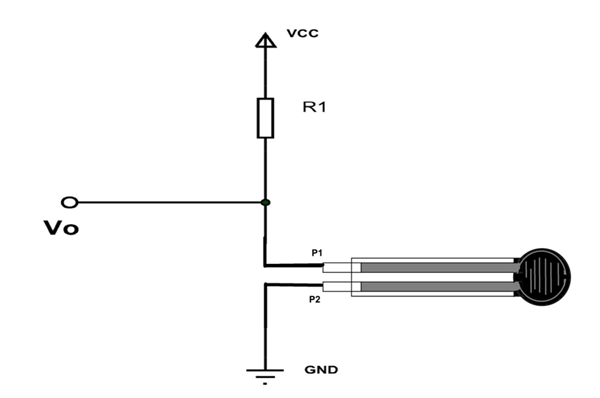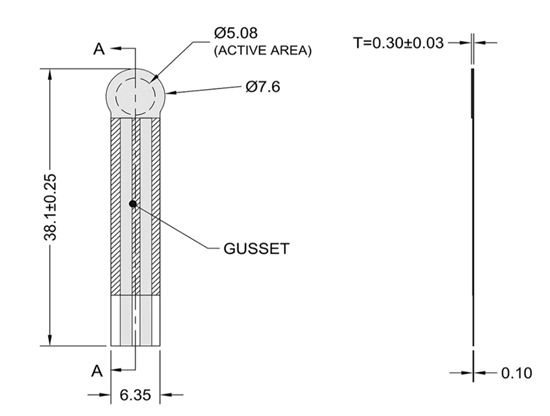# FSR400 - Force Sensor

[Click the image to enlarge it]

Force sensors are available in different sizes and shapes. Although the sizes and shapes are different the basic function remains the same. You can choose the appropriate type depending on the requirements.  Here we are going to discuss 38mm Pressure Sensor or Force Sensor FSR400.

FORCE SENSOR Function: FORCE SENSOR terminal resistance decreases when pressure is applied on the surface.

### FORCE SENSOR Pin Configuration

FORCE sensor is a two terminal device. The Force sensor does not have polarized terminals like diode. So there is no positive and negative.

 Pin Number Description P1 Terminal one of sensor P2 Terminal two of sensor

### FORCE SENSOR Features and Specifications

• Actuation force as low as 0.2N
• Sensitivity range up to 20N
• Cost effective
• Ultra thin
• Life: 10 million
• Simple and easy to integrate
• Operating temperature: -40ºC to +85ºC
• Non actuated  Resistance: 10M Ω
• Device rise time: < 3 Micro second
• Bend Resistance Range: 100K to 1K Ohms(depending on force)

### FSR400 Equivalents

FSR402, FSR406, FSR408

### Where FSR400 FORCE SENSORS are used?

There are many reasons why FSR400 is preferred here are a few

Case1: FSR400 is one of the cheapest force sensors on the market. They are easily available.

Case2:  FSR400 is very easy to use sensor module. There is no additional equipment needed for installing the sensor in applications.

Case3: FSR400 is very portable and can be used very conveniently

Case4: This sensor has a very long life and so can be installed in permanently fixed system.

With very fast response and other features usage of FSR400 is further promoted as force sensing device.

### How to Use FSR400 FORCE SENSOR

As mentioned earlier, FORCE SENSOR terminal resistance decreases when pressure is applied on the surface of the sensor. So force sensor is basically a VARIABLE RESISTOR whose terminal resistance depends of surface pressure.

With higher pressure the terminal resistance will be low and for low pressure resistance will be high. So in a sense the force sensor converts pressure to inverted RESISTANCE parameter.

For convenience we convert this RESISTANCE parameter to VOLTAGE parameter. For that we are going to use VOLTAGE DIVIDER circuit.

In this resistive network we have two resistances. One is constant resistance (R1) and other is variable resistance (RV1). Vo is the voltage at midpoint of VOLTAGE DIVIDER circuit and is also the output voltage. Vo is also the voltage across the variable resistance (RV1). So when the resistance value of RV1 is changed the output voltage Vo also changes. So we will have resistance change in voltage change with VOLTAGE DIVIDER circuit.

The circuit of force sensor with voltage divider is shown below.Here R1 here is a constant resistance and FORCE SENSOR acts as a variable resistance. Vo being output voltage and also the voltage across the FORCE SENSOR.

Here, Vo = VCC (Rx/ (R1+Rx)).

Rx - FORCE SENSOR resistance

Now, when there is no pressure applied on FORCE SENSOR the terminal resistance will be very high. This resistance also appears in VOLTAGE DIVIDER circuit. So the total voltage VCC appears across sensor.  With that Vo will be high as it is the voltage across sensor.

When the pressure is applied on the surface of sensor the terminal resistance decreases considerable. With this decrease the voltage drop across sensor in the voltage divider circuit also decreases. So Vo also goes down. If pressure further increased the Vo further decreases.

Here we can see the relationship between applied pressure and Vo. With that we have VOLTAGE parameter (Vo) representing the pressure.

We can take this VOLTAGE parameter and feed it to ADC to get the digital value which can be used conveniently.

### Applications

• Robotics
• Detect motion or position
• Medical Devices
• Detect and qualify press
• Find center of force
• Detect pressure
• Detect liquid blockage

### 2D-Model

Measurements in millimeterComponent Datasheet